# SSD学习笔记

## 1. SSD300的结构：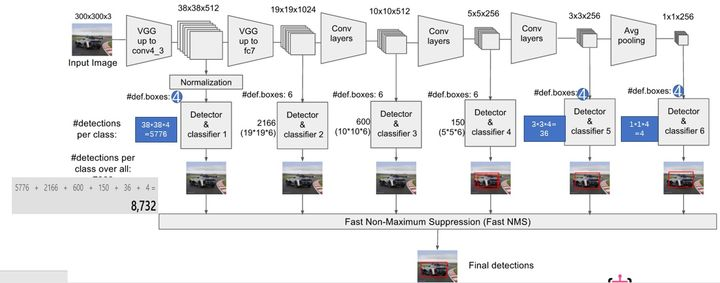Base network and hole filling algorithm

https://blog.csdn.net/u010167269/article/details/52563573

ICLR 2015, DeepLab-LargeFOV 的工作类似，*本文将 VGG 中的 FC6 layer、FC7 layer 转成为 卷积层，并从模型的 FC6、FC7 上的参数进行采样得到这两个卷积层的 parameters。*本文还将 fully convolutional reduced (atrous) VGGNet 中的所有的 dropout layers、fc8 layer 移除掉了。

pool4 的 stride 由 2 变为 1，则紧接着的 conv5_1, conv5_2 和 conv5_3 中 hole size 为 2。接着 pool5 由 2 变为 1 , 则后面的 fc6 中 hole size 为 4。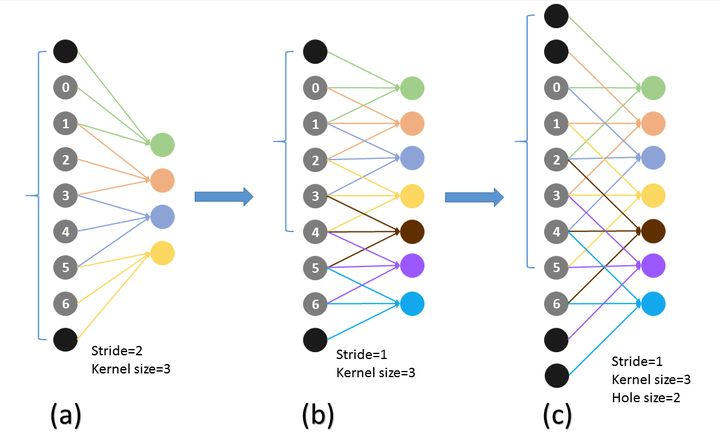## 2. SSD训练时的ground truth box和default box的匹配原则：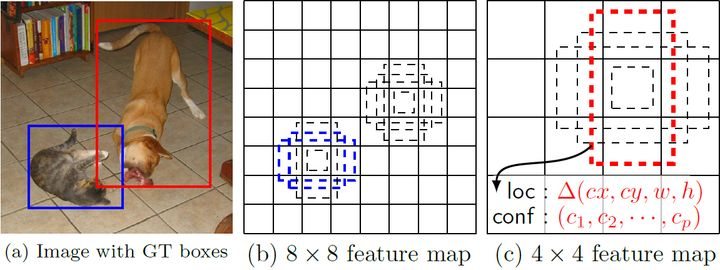*(1)***首先，对于图片中每个ground truth，找到与其IOU最大的先验框，该先验框与其匹配，这样，可以保证每个ground truth一定与某个先验框匹配。**通常称与ground truth匹配的先验框为正样本（其实应该是先验框对应的预测box，不过由于是一一对应的就这样称呼了），**反之，若一个先验框没有与任何ground truth进行匹配，那么该先验框只能与背景匹配，就是负样本。**一个图片中ground truth是非常少的， 而先验框却很多，如果仅按第一个原则匹配，很多先验框会是负样本，正负样本极其不平衡，所以需要第二个原则。

(2)第二个原则是：对于剩余的未匹配先验框，若某个ground truth的 IOU \text{IOU} 大于阈值，那么先验框只与IOU最大的那个先验框进行匹配。第二个原则一定在第一个原则之后进行，仔细考虑一下这种情况，如果某个ground truth所对应最大 IOU \text{IOU} 小于阈值，并且所匹配的先验框却与另外一个ground truth的 IOU \text{IOU} 大于阈值，那么该先验框应该匹配谁，答案应该是前者，首先要确保某个ground truth一定有一个先验框与之匹配。但是，这种情况我觉得基本上是不存在的。由于先验框很多，某个ground truth的最大 IOU \text{IOU} 肯定大于阈值，所以可能只实施第二个原则既可以了，这里的TensorFlow版本就是只实施了第二个原则，但是这里的Pytorch两个原则都实施了。图8为一个匹配示意图，其中绿色的GT是ground truth，红色为先验框，FP表示负样本，TP表示正样本。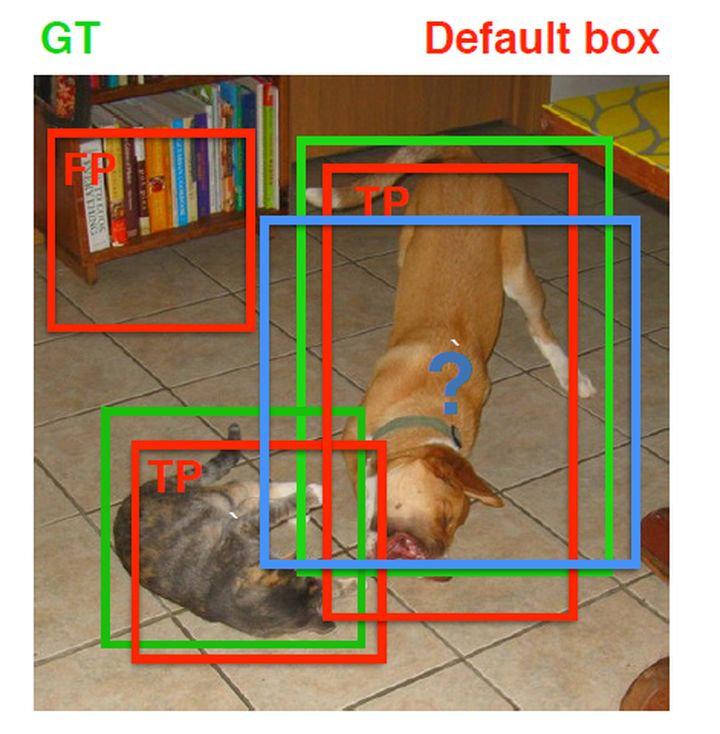## 3. SSD代码中variance超参数的作用：

The meanings of parameter “variance” in PriorBox layer · Issue #75 · weiliu89/caffe

https://blog.csdn.net/Suodislie/article/details/79607489

It is used to encode the ground truth box w.r.t. the prior box. You can check this function. Note that it is used in the original MultiBox paper by Erhan etal. It is also used in Faster R-CNN as well. I think the major goal of including the variance is to scale the gradient. Of course you can also think of it as approximate a gaussian distribution with variance of 0.1 around the box coordinates.

## 4. 不同阶段feature map设置不同scale的default box的原因：

anchor boxes(default boxes)的size是我们人为固定好了的（anchor boxes的大小对应的是原图像的，它中心点是原图像上anchor boxes的中心点，对应到示意图里就是那个蓝点点）。ground truth boxes要和映射到原图像中的default box求IOU的。但是实际上神经网络中并没有把这k个anchor boxed坐标当做神经网络里的参数。而是，神经网络在训练的过程中，每个anchor会根据它对应的k个boxes学出4*k个坐标矫正量（坐标矫正量是根据，“anchor对应的anchor boxes的坐标物体的ground truth的坐标，这二者的偏差量”得到的loss来学习）。faster rcnn中anchor的好处是稳定训练过程，因为这么多anchor中已经有和物体iou比较大的了(也就是说坐标值是比较接近了，这样做offset能够更简单)，如果一上来就直接回归box，很可能不收敛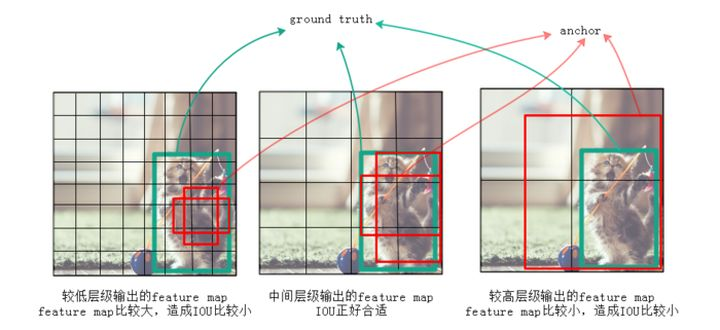## 5. Default Box的尺寸设计：

CSDN的一个博客做了详细的分析：

SSD详解Default box的解读blog.csdn.net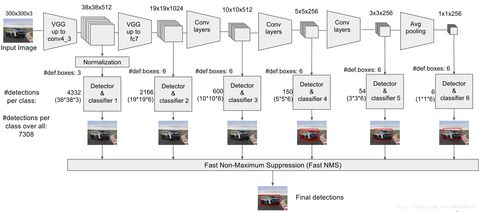prior box是按照不同的 scale 和 ratio 生成，k (默认是6，但是有的层不一定，比如conv4_3层的是3)个 default boxes，这种结构有点类似于 Faster R-CNN 中的 Anchor。(此处k=6所以： 5 × 5 × 6 = 150 b o x e s 5\times 5\times 6 = 150 boxes )。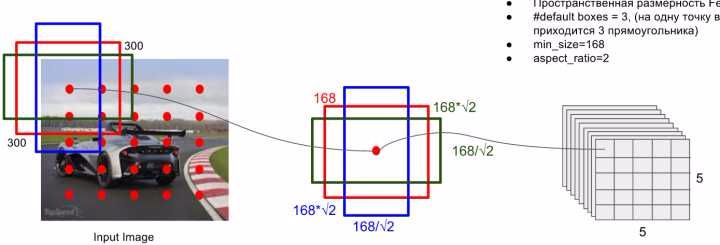default box示意图

• scale: 假定使用N个不同层的feature map 来做预测。最底层的 feature map 的 scale 值为 s m i n = 0.2 s_{min}=0.2 ，最高层的为 s m a x = 0.9 s_{max}=0.9 ，其他层通过下面公式计算得到 s k = s m i n + s m a x − s m i n m − 1 ( k − 1 ) , k ∈ [ 1 , N ] s_k = s_{min}+\frac{s_{max}-s_{min}}{m-1}(k-1), k\in [1,N] (低层检测小目标，高层检测大目标)。当前300×3×3网络一共使用了6(N=6)个feature map，即网络结构图中的detector1…detector6。比如第一层detector1的sk=0.2，第二层的detector2 s k = 0.2 + 0.9 − 0.2 6 − 1 ( 2 − 1 ) = 0.34 s_k=0.2+\frac{0.9-0.2}{6-1}(2-1)=0.34 ,…第五层detector5 s k = 0.2 + 0.9 − 0.2 6 − 1 ( 5 − 1 ) = 0.76 s_k=0.2+\frac{0.9-0.2}{6-1}(5-1)=0.76
• ratio: 使用不同的 ratio值ar∈{1,2,1/2,3,1/3} 计算 default box 的宽度和高度： w K a = s k a r , h k a = s k / a r w_K^{a} = s_k \sqrt{a_r} , h_k^{a} =s_k/\sqrt{a_r} 。另外对于 ratio = 1 的情况，额外再指定 scale 为 s k ‘ = s k s k + 1 s_k{`}=\sqrt{s_ks_{k+1}} 也就是总共有 6 中不同的 default box。比如示意图中的为detector4，其sk=0.62,依据公式 w K a = s k a r w_K^{a} = s_k \sqrt{a_r} 按照{1,2,1/2,3,1/3}顺序可以有 w k a w_k^a : [ 0.62 × 300 , 0.62 × 1.414 × 300 , 0.62 × 0.707 × 300 , 0.62 × 1.732 × 300 , 0.62 × 0.577 × 300 ] [0.62\times300,0.62\times1.414\times300,0.62\times0.707\times300,0.62\times1.732\times300,0.62\times0.577\times300] 与图中的168不一致
• default box中心：上每个 default box的中心位置设置成(i+0.5|fk|,j+0.5|fk|) ，其中 |fk| 表示第k个特征图的大小i,j∈[0,|fk|] 。

## 6. SSD中有且只有conv4_3层做了L2归一化

Normalize 操作很简单：给定一个向量x, 对它进行 $Lp$ normalization操作是指对它的元素值进行放缩: ，得到的 x ~ \tilde{x} 的 $Lp$ norm值刚好为1， 其中 L p n o r m ( x ) = ( ∑ i x i p ) 1 p Lp norm(x)=\left(\sum_{i}^{}{x_{i}^{p}} \right)^{\frac{1}{p}} . 在训练深度模型的过程中， 加入normalization操作通常可以增加算法的鲁棒性，类似的操作还有BN（BatchNormalization）. 但如果把feature的模长放缩到刚好等于一的长度，会让学到的feature变得很小，网络会难以训练。所以，更可取的做法是将feature的值放大一定倍数，例如20倍，这就是scale_filler的作用 x ~ ← s c a l e ∗ x ~ \tilde{x}\leftarrow scale*\tilde{x} . across_spatial如果为true的话，则按channel进行norm操作。 此处为false，被norm的是像素的feature vector。例如，现在要对大小为m×n×c的feature map进行norm操作，前者是在c个m×n的tensor进行norm操作，后者在m×n个c维向量上操作。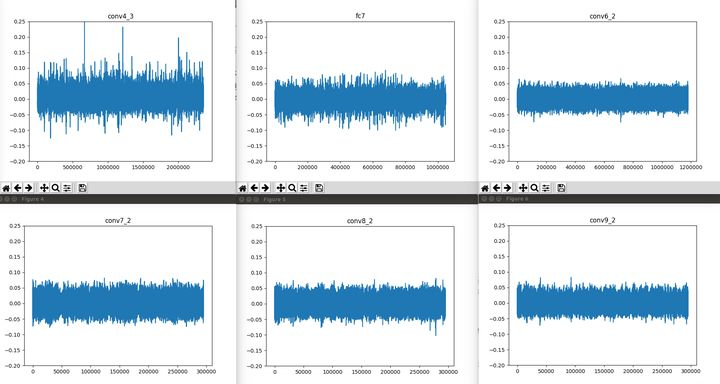VGG不同特征图权值的数值分布图

## 7. SSD中位置回归的形式

SSD的检测值也与Yolo不太一样。对于每个单元的每个先验框，其都输出一套独立的检测值，对应一个边界框，主要分为两个部分。第一部分是各个类别的置信度或者评分，值得注意的是SSD将背景也当做了一个特殊的类别，如果检测目标共有 c c 个类别，SSD其实需要预测 c + 1 c+1 个置信度值，其中第一个置信度指的是不含目标或者属于背景的评分。后面当我们说 c c 个类别置信度时，请记住里面包含背景那个特殊的类别，即真实的检测类别只有 c − 1 c-1 个。在预测过程中，置信度最高的那个类别就是边界框所属的类别，特别地，当第一个置信度值最高时，表示边界框中并不包含目标。第二部分就是边界框的location，包含4个值 ( c x , c y , w , h ) (cx, cy, w, h) ，分别表示边界框的中心坐标以及宽高。但是真实预测值其实只是边界框相对于先验框的转换值(paper里面说是offset，但是觉得transformation更合适，参见R-CNN)。先验框位置用 d = ( d c x , d c y , d w , d h ) d=(d^{cx}, d^{cy}, d^w, d^h) 表示，其对应边界框用 b = ( b c x , b c y , b w , b h ) b=(b^{cx}, b^{cy}, b^w, b^h) \$表示，那么边界框的预测值 l l 其实是 b b 相对于 d d 的转换值：

l c x = ( b c x − d c x ) / d w ,   l c y = ( b c y − d c y ) / d h l^{cx} = (b^{cx} - d^{cx})/d^w, \space l^{cy} = (b^{cy} - d^{cy})/d^h

l w = log ⁡ ( b w / d w ) ,   l h = log ⁡ ( b h / d h ) l^{w} = \log(b^{w}/d^w), \space l^{h} = \log(b^{h}/d^h)

b c x = d w l c x + d c x ,   b c y = d y l c y + d c y b^{cx}=d^w l^{cx} + d^{cx}, \space b^{cy}=d^y l^{cy} + d^{cy}

b w = d w exp ⁡ ( l w ) ,   b h = d h exp ⁡ ( l h ) b^{w}=d^w \exp(l^{w}), \space b^{h}=d^h \exp(l^{h})

b c x = d w ( v a r i a n c e [ 0 ] ∗ l c x ) + d c x ,   b c y = d y ( v a r i a n c e [ 1 ] ∗ l c y ) + d c y b^{cx}=d^w (variance*l^{cx}) + d^{cx}, \space b^{cy}=d^y (variance*l^{cy}) + d^{cy}

b w = d w exp ⁡ ( v a r i a n c e [ 2 ] ∗ l w ) ,   b h = d h exp ⁡ ( v a r i a n c e [ 3 ] ∗ l h ) b^{w}=d^w \exp(variance*l^{w}), \space b^{h}=d^h \exp(variance*l^{h})

10-1708-121110
12-297651
10-14166
05-30
04-03482
05-223万+
08-08963
03-192100
08-09386
03-3068
10-1795
12-223万+
01-27198
12-051013
08-07555
09-199万+
02-203328
05-121315
©️2020 CSDN 皮肤主题: 编程工作室 设计师:CSDN官方博客